# 三、使用类处理图像

• 在算法设计中使用策略模式
• 使用控制器与处理模块通信
• 使用单例设计模式
• 使用模型-视图-控制器架构设计应用
• 转换色彩空间

# 在策略设计中使用策略模式

## 操作步骤

     // get the iterators
cv::Mat_<cv::Vec3b>::const_iterator it=
image.begin<cv::Vec3b>();
cv::Mat_<cv::Vec3b>::const_iterator itend=
image.end<cv::Vec3b>();
cv::Mat_<uchar>::iterator itout=
result.begin<uchar>();

// for each pixel
for ( ; it!= itend; ++it, ++itout) {

// process each pixel ---------------------

// compute distance from target color
if (getDistance(*it)<minDist) {

*itout= 255;

} else {

*itout= 0;
}

// end of pixel processing ----------------
}


cv::Mat变量image表示输入图像，而result表示二进制输出图像。 因此，第一步包括设置所需的迭代器。 这样，即可轻松实现扫描for循环。 每次迭代都会检查当前像素颜色和目标颜色之间的距离是否在minDist定义的公差范围内。 如果是这种情况，则将值255（白色）分配给输出图像，如果不是，则分配0（黑色）。 要计算两个颜色值之间的距离，请使用getDistance方法。 有多种计算此距离的方法。 例如，可以计算包含 RGB 颜色值的 3 个向量之间的欧式距离。 在我们的案例中，为使计算简单有效，我们简单地将 RGB 值的绝对差求和（也称为城市街区距离）。 getDistance方法的定义如下：

     // Computes the distance from target color.
int getDistance(const cv::Vec3b& color) const {

return abs(color-target)+
abs(color-target)+
abs(color-target);
}


cv::Mat ColorDetector::process(const cv::Mat &image) {

// re-allocate binary map if necessary
// same size as input image, but 1-channel
result.create(image.rows,image.cols,CV_8U);

*processing loop above goes here*
...

return result;
}


class ColorDetector {

private:

// minimum acceptable distance
int minDist;

// target color
cv::Vec3b target;

// image containing resulting binary map
cv::Mat result;


     // empty constructor
ColorDetector() : minDist(100) {

// default parameter initialization here
target= target= target= 0;
}


     // Sets the color distance threshold.
// Threshold must be positive,
// otherwise distance threshold is set to 0.
void setColorDistanceThreshold(int distance) {

if (distance<0)
distance=0;
minDist= distance;
}

// Gets the color distance threshold
int getColorDistanceThreshold() const {

return minDist;
}


     // Sets the color to be detected
void setTargetColor(unsigned char red,
unsigned char green,
unsigned char blue) {

// BGR order
target= red;
target= green;
target= blue;
}

// Sets the color to be detected
void setTargetColor(cv::Vec3b color) {

target= color;
}

// Gets the color to be detected
cv::Vec3b getTargetColor() const {

return target;
}


## 工作原理

int main()
{
// 1\. Create image processor object
ColorDetector cdetect;

if (!image.data)
return 0;

// 3\. Set input parameters
cdetect.setTargetColor(130,190,230); // here blue sky

cv::namedWindow("result");

// 4\. Process the image and display the result
cv::imshow("result",cdetect.process(image));

cv::waitKey();

return 0;
}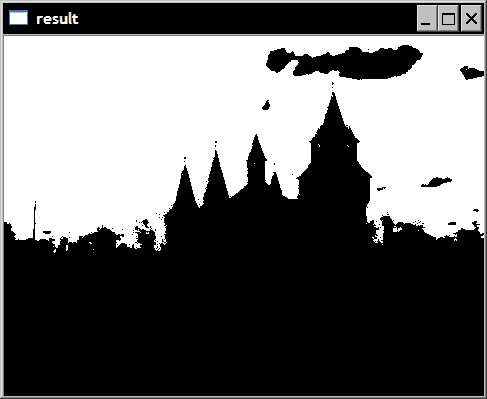## 更多

return abs(color-target)+
abs(color-target)+
abs(color-target);


return static_cast<int>(
cv::norm<int,3>(cv::Vec3i(color-target,
color-target,
color-target)));


c= a+b;


return static_cast<int>(
cv::norm<uchar,3>(color-target);


## 另见

A. Alexandrescu 引入的基于策略的类设计是策略设计模式的一个有趣变体，其中在编译时选择算法。

# 使用控制器与处理模块通信

## 准备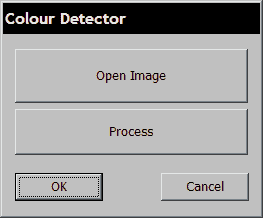## 操作步骤

class ColorDetectController {

private:

// the algorithm class
ColorDetector *cdetect;

cv::Mat image;   // The image to be processed
cv::Mat result;  // The image result

public:

ColorDetectController() {

//setting up the application
cdetect= new ColorDetector();
}


     // Sets the color distance threshold
void setColorDistanceThreshold(int distance) {

cdetect->setColorDistanceThreshold(distance);
}

// Gets the color distance threshold
int getColorDistanceThreshold() const {

return cdetect->getColorDistanceThreshold();
}

// Sets the color to be detected
void setTargetColor(unsigned char red,
unsigned char green, unsigned char blue) {

cdetect->setTargetColor(red,green,blue);
}

// Gets the color to be detected
void getTargetColor(unsigned char &red,
unsigned char &green, unsigned char &blue) const {

cv::Vec3b color= cdetect->getTargetColor();

red= color;
green= color;
blue= color;
}

// Sets the input image. Reads it from file.
bool setInputImage(std::string filename) {

if (!image.data)
return false;
else
return true;
}

// Returns the current input image.
const cv::Mat getInputImage() const {

return image;
}


     // Performs image processing.
void process() {

result= cdetect->process(image);
}


     // Returns the image result from the latest processing.
const cv::Mat getLastResult() const {

return result;
}


     // Deletes processor objects created by the controller.
~ColorDetectController() {

delete cdetect;
}


## 工作原理

// Callback method of "Open" button.
void OnOpen()
{
// MFC widget to select a file of type bmp or jpg
CFileDialog dlg(TRUE, _T("*.bmp"), NULL,
_T("image files (*.bmp; *.jpg)
|*.bmp;*.jpg|All Files (*.*)|*.*||"),NULL);

dlg.m_ofn.lpstrTitle= _T("Open Image");

// if a filename has been selected
if (dlg.DoModal() == IDOK) {

// get the path of the selected filename
std::string filename= dlg.GetPathName();

// set and display the input image
colordetect.setInputImage(filename);
cv::imshow("Input Image",colordetect.getInputImage());
}
}


// Callback method of "Process" button.
void OnProcess()
{
// target color is hard-coded here
colordetect.setTargetColor(130,190,230);

// process the input image and display result
colordetect.process();
cv::imshow("Output Result",colordetect.getLastResult());
}


# 使用单例设计模式

## 操作步骤

   class ColorDetectController {

private:

// pointer to the singleton
static ColorDetectController *singleton;

ColorDetector *cdetect;

// private constructor
ColorDetectController() {

//setting up the application
cdetect= new ColorDetector();
}


     // Gets access to Singleton instance
static ColorDetectController *getInstance() {

// Creates the instance at first call
if (singleton == 0)
singleton= new ColorDetectController;

return singleton;
}


     // Releases the singleton instance of this controller.
static void destroy() {

if (singleton != 0) {
delete singleton;
singleton= 0;
}
}


#include "colorDetectController.h"

ColorDetectController *ColorDetectController::singleton=0;


## 工作原理

// Callback method of "Open" button.
void OnOpen()
{
...

// if a filename has beed selected
if (dlg.DoModal() == IDOK) {

// get the path of the selected filename
std::string filename= dlg.GetPathName();

// set and display the input image
ColorDetectController::
getInstance()->setInputImage(filename);
cv::imshow("Input Image",
ColorDetectController::
getInstance()->getInputImage());
}
}

// Callback method of "Process" button.
OnProcess()
{
// target color is hard-coded here
ColorDetectController::
getInstance()->setTargetColor(130,190,230);

// process the input image and display result
ColorDetectController::getInstance()->process();
cv::imshow("Output Result",
ColorDetectController::getInstance()->getLastResult());
}


// Callback method of "Close" button.
void OnClose()
{
// Releases the Singleton.
ColorDetectController::getInstance()->destroy();
OnOK();
}


# 使用模型-视图-控制器架构设计应用

## 操作步骤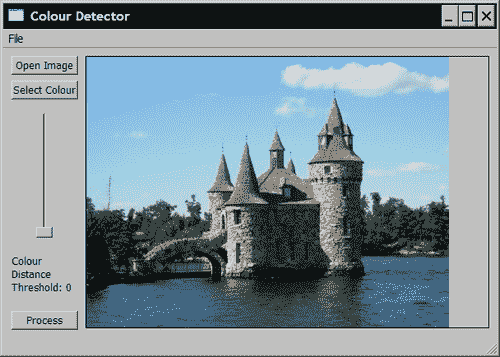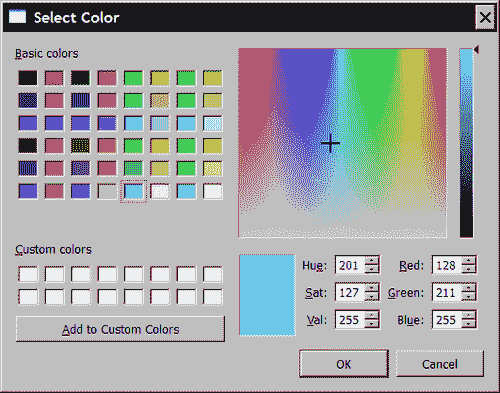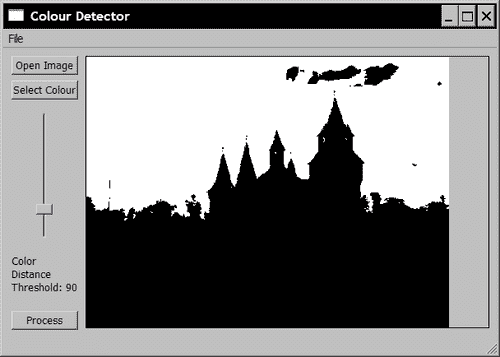## 工作原理

QColor color = QColorDialog::getColor(Qt::green, this);
if (color.isValid()) {
ColorDetectController::getInstance()
->setTargetColor(color.red(),color.green(),color.blue());
}


ColorDetectController::getInstance()
->setColorDistanceThreshold(
ui->verticalSlider_Threshold->value());
ColorDetectController::getInstance()->process();
cv::Mat resulting =
ColorDetectController::getInstance()->getLastResult();
if (!resulting.empty())
displayMat(resulting);


# 转换色彩空间

## 准备

RGB 颜色空间（或 BGR，取决于存储颜色的顺序）基于红色，绿色和蓝色加法原色的使用。 之所以选择这些是因为将它们组合在一起可以产生各种颜色的色域。 实际上，人类视觉系统还基于三色感知的颜色，视锥细胞敏感度位于红色，绿色和蓝色光谱附近。 它通常是数字图像中的默认色彩空间，因为这是获取色彩的方式。 捕获的光通过红色，绿色和蓝色过滤器。 另外，在数字图像中，调节红色，绿色和蓝色通道，使得当以等量组合时，获得灰度级强度，即从黑色(0,0,0)到白色(255,255,255)

## 操作步骤

cv::Mat ColorDetector::process(const cv::Mat &image) {

// re-allocate binary map if necessary
// same size as input image, but 1-channel
result.create(image.rows,image.cols,CV_8U);

// re-allocate intermediate image if necessary
converted.create(image.rows,image.cols,image.type());

// Converting to Lab color space
cv::cvtColor(image, converted, CV_BGR2Lab);

// get the iterators of the converted image
cv::Mat_<cv::Vec3b>::iterator it=
converted.begin<cv::Vec3b>();
cv::Mat_<cv::Vec3b>::iterator itend=
converted.end<cv::Vec3b>();
// get the iterator of the output image
cv::Mat_<uchar>::iterator itout= result.begin<uchar>();

// for each pixel
for ( ; it!= itend; ++it, ++itout) {
...


class ColorDetector {

private:
// image containing color converted image
cv::Mat converted;


     // Sets the color to be detected
void setTargetColor(unsigned char red,
unsigned char green, unsigned char blue) {

// Temporary 1-pixel image
cv::Mat tmp(1,1,CV_8UC3);
tmp.at<cv::Vec3b>(0,0)= blue;
tmp.at<cv::Vec3b>(0,0)= green;
tmp.at<cv::Vec3b>(0,0)= red;

// Converting the target to Lab color space
cv::cvtColor(tmp, tmp, CV_BGR2Lab);

target= tmp.at<cv::Vec3b>(0,0);
}


## 工作原理

HSV 和 HLS 颜色空间也很有趣，因为它们将颜色分解为其色相和饱和度分量以及值或亮度分量，这是人类描述颜色的一种更自然的方式。

         cv::cvtColor(color, gray, CV_BGR2Gray);